MathematicsAnalyzing Residuals

A measure of the "goodness" of fit is the residual, the difference between the observed and predicted data. Compare the residuals for the various fits, using normalized `cdate` values. It's evident from studying the fit plots and residuals that it should be possible to do better than a simple polynomial fit with this data set.

 Fit Residuals `p1 = polyfit(sdate,pop,1);``pop1 = polyval(p1,sdate);``plot(cdate,pop1,'b-',cdate,pop,'g+')`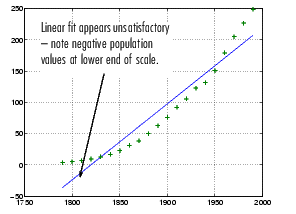`res1 = pop - pop1;``figure, plot(cdate,res1,'g+')`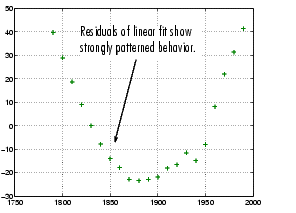`p = polyfit(sdate,pop,2);``pop2 = polyval(p,sdate);``plot(cdate,pop2,'b-',cdate,pop,'g+')`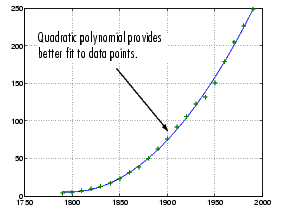`res2 = pop - pop2;``figure, plot(cdate,res2,'g+')`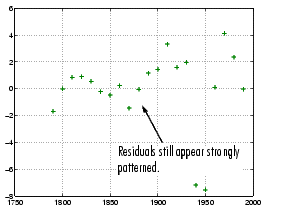`p = polyfit(sdate,pop,4);``pop4 = polyval(p,sdate);``plot(cdate,pop4,'b-',cdate,pop,'g+')`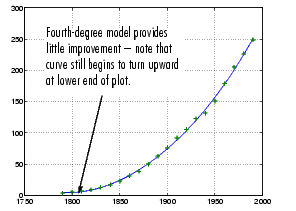`res4 = pop - pop4;``figure, plot(cdate,res4,'g+')`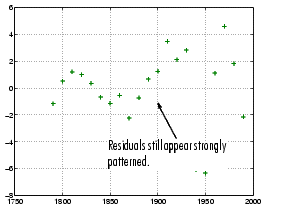Polynomial Fit Exponential Fit© 1994-2005 The MathWorks, Inc.